Cups

We have three cups. In the cups we had fluid and boredom we started to shed.

1 We shed one-third of the fluid from the second glass into the first and third.
2 Then we shed one quarter cup of liquid from the first to the second and to the third.
3 Then we shed one-fifth of the liquid from the third to the first cup and to the second.

Finally remained 8, 10 and 7 dl fluids in cups. How many dl of liquid was in the cups originally?

Result

a =  6.5 dl
b =  14.5 dl
c =  4 dl

Solution:

d=a+b/3
e=b/3
f = c+b/3
k = d/2
l = e+d/4
m = f+d/4
x = k+m/5
y = l+m/5
z = m *3/5
x=8
y=10
z = 7

3a+b-3d = 0
b-3e = 0
b+3c-3f = 0
d-2k = 0
d+4e-4l = 0
d+4f-4m = 0
5k+m-5x = 0
5l+m-5y = 0
3m-5z = 0
x = 8
y = 10
z = 7

a = 132 = 6.5
b = 292 = 14.5
c = 4
d = 343 ≈ 11.333333
e = 296 ≈ 4.833333
f = 536 ≈ 8.833333
k = 173 ≈ 5.666667
l = 233 ≈ 7.666667
m = 353 ≈ 11.666667
x = 8
y = 10
z = 7

Calculated by our linear equations calculator.

Leave us a comment of this math problem and its solution (i.e. if it is still somewhat unclear...):Be the first to comment!To solve this verbal math problem are needed these knowledge from mathematics:

Need help calculate sum, simplify or multiply fractions? Try our fraction calculator. Do you have a system of equations and looking for calculator system of linear equations?

Next similar math problems:

1. Three brothersThe three brothers have a total of 42 years. Jan is five years younger than Peter and Peter is 2 years younger than Michael. How many years has each of them?
2. Two numbersWe have two numbers. Their sum is 140. One-fifth of the first number is equal to half the second number. Determine those unknown numbers.
3. Waiting roomIn the waiting room are people and flies. Together they have 15 heads and 50 legs (fly has 6 legs). How many people and flies are in the waiting room?
4. Sisters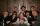Sisters Janka and Danka are saved together 220 CZK. On a trip to Janka wants to take a fifth of her savings and Danka quarter. Then they will have 50 CZK on the trip. How much money saved Janka and Danka?
5. Three men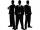Alex is half younger than Jan, which is one-third younger than George. The sum of their ages is 48. How are these three men old?
6. Hotel roomsIn the 45 rooms, there were 169 guests, some rooms were three-bedrooms and some five-bedrooms. How many rooms were?
7. Tram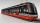In the three-part tram went 206 passengers in front of others, 226 after others and in the middle half of all. How many passengers went in tram total?
8. EquationsSolve following system of equations: 6(x+7)+4(y-5)=12 2(x+y)-3(-2x+4y)=-44
9. TheatroTheatrical performance was attended by 480 spectators. Women were in the audience 40 more than men and children 60 less than half of adult spectators. How many men, women and children attended a theater performance?
10. Equations - simpleSolve system of linear equations: x-2y=6 3x+2y=4
11. The dormitoryThe dormitory accommodates 150 pupils in 42 rooms, some of which are triple and some are quadruple. Determine how many rooms are triple and how many quadruples.
12. MushroomsEva and Jane collected 114 mushrooms together. Eve found twice as much as Jane. How many mushrooms found each of them?
13. TreesAlong the road were planted 250 trees of two types. Cherry for 60 CZK apiece and apple 50 CZK apiece. The entire plantation cost 12,800 CZK. How many was cherries and apples?
14. Linear systemSolve this linear system (two linear equations with two unknowns): x+y =36 19x+22y=720
15. Brunette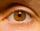Of the girls who attend primary school are 30% blondes and 70% brunette. 81% blondes and 19% brunette has blue eyes. How many girls attending school altogether if 470 girls has blue eyes?
16. Donuts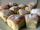In the dining room at one table sit Thomas, Filip and Martin. Together they eat 36 donuts. How many donuts eaten everyone, when Filip ate twice as much as Thomas and Martin even half over Filip?
17. Pages of book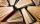Lenka calculated that if every day to read 16 pages of the book, read a book a day earlier than had read only 14 pages a day. How many pages has this book?